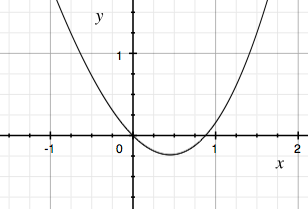SEARCH HOMEMath Central Quandaries & QueriesQuestion from Kemboi, a student: Find the positive root of the equation sin(x) = x^2Hi Kemboi,

The subject line of your email to us was "Newton Raphson" so I expect you are to use this method to approximate the root. I would first let $f(x) = x^2 - sin(x)$ and apply the Newton Raphson method as I did in my response to an earlier question. As is mentioned there one of the challenges is to find an appropriate starting value $x_{0}.$ Since you used a computer to send your question I assume you have access to a graphing program. I used Grapher on my Mac and got the following for the graph of $y = x^2 - sin(x).$From the graph it seems that $x = 0.8$ radians is close to the root you want so I would take $x_0 = 0.8$ radians.

PennyMath Central is supported by the University of Regina and the Imperial Oil Foundation.